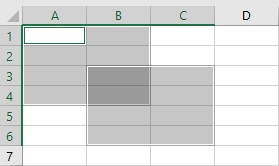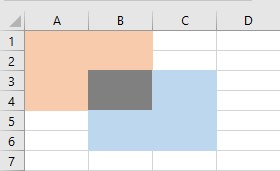# Excel VBA – Union and Intersect

Excel VBA has two methods, belonging to Application object, to manipulate two or more ranges: Union and Intersect.

## Union

Union method returns all the cells in two or more ranges passed as its argument.

The following command will select the range shown in the image below:

``Union(Range("A1:B4"),Range("B3:C6")).Select``You can assign any value or formula to the range returned by the Union method:

``Union(Range("A1:B4"), Range("B3:C6")) = 10``

This will enter the value 10 in each cell in the Union.

You can wrap any function which summarizes a range around an Union method. Following example will return the sum of the values in the Ranges A1:B4 and B3:C6:

``Result = Application.WorksheetFunction.Sum(union(Range("A1:B4"), Range("B3:C6")))``

You might be surprised to get the value in Result as 160! Although there are only 14 cells in the Union (8 in each range with 2 being common) when you look at Selection, Union actually returns 16 cells hence the Result as 160.

## Intersect

Intersect method returns only the common cells in two or more ranges passed as its argument.

The following command will select the range shown (Gray area) in the image below:

``Intersect(Range("A1:B4"),Range("B3:C6")).Select``## Use of Intersect

The most common usage of Intersect is in events associated with a Worksheet or Workbook. It is used to test whether the cell(s) changed belong to a a range of interest. Following example with check whether the cell(s) changed (identified by Target) and Range A1:A10  are common and take appropriate action if they are.

Intersect object returns nothing if there are no common cells so Intersect(Target, Range(“A1:A10”)) Is Nothing will be True if there are no common cells. Adding Not to the condition makes it True only if the result of the test Intersect(Target, Range(“A1:A10”)) Is Nothing is False, in other words Target and Range A1:A10 have some cells in common.

``````Private Sub Worksheet_Change(ByVal Target As Range)
If Not Intersect(Target, Range("A1:A10")) Is Nothing Then
' Take desired action
End If
End Sub``````

Written by: Vinamra Chandra

Stop searching for VBA code online. Learn more about AutoMacro - A VBA Code Builder that allows beginners to code procedures from scratch with minimal coding knowledge and with many time-saving features for all users!